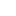# How to Estimate Strength of Concrete through Maturity method? [PDF]Reading time: 1 minute

The maturity of concrete is determined by a non-destructive approach, where the compressive strength at early ages is detected to conclude about the maturity. This test is conducted on an in-place real-time concrete mix at the site. The use of a maturity test on concrete at the job site helps to eliminate the need for concrete cylinder breaking tests and hence saves and optimizes the project schedule.

## Concrete Maturity and Maturity Method on Concrete

The maturity method follows the concept that the strength of concrete and other related properties are directly proportional to the age and the temperature variations of the concrete structure.

The maturity method determines the compressive strength and flexural strength of concrete at an early age (in-place concrete) of construction. The principle followed is that the concrete samples with the same maturity possess similar strength irrespective of the time and temperature combinations that yield the maturity.

The maturity of concrete is measured in terms of the “maturity index” of the in-place concrete. The Maturity Index is a function of age and the history of temperature variations of the concrete.

The obtained value of maturity index is used to study a standard graphical representation – Strength-Maturity Relationship Graph, from which the strength of concrete is estimated. The strength-maturity relationship graph is obtained by conducting a standard laboratory test for a given concrete mixture.

## Principle of Maturity Method for Concrete Testing

The concrete attains the properties during the hydration process (with the release of heat). The early-stage strength development of the concrete is related to the rate of hydration of the concrete.

During the concrete hydration process, the heat of hydration generated is measured in terms of the rate of temperature rise in the concrete specimen. This way, the maturity method employs the actual temperature of the concrete to analyze the concrete strength at the site. In traditional methods, the exact temperature is not used, which affects the accuracy of the strength value of the concrete.

The maturity method hence provides the real-time strength value of the concrete at the site at a particular time. This way, optimization of workflow is attained, thus achieving more accurate in-place strength.

## Maturity Method Steps

The basic steps involved in the maturity method testing on in-place concrete are:

1. Track the internal temperatures of the concrete specimen during the curing process.
2. A concrete maturity sensor can be used to measure the concrete temperature and maturity value. Maturity can also be calculated from the history of tracked internal temperatures.
3. Strength is estimated from the strength-maturity relationship that is standardized.

## Advantages of Maturity Method Test on Concrete

The main advantages of conducting maturity method for concrete testing are:

• The strength of concrete is determined within a lesser time period.
• The real-time concrete strength is determined.
• The number of test specimens required to conduct quality control (QC) and quality assurance (QA) decreases.
• In prestressed structures, the stressing of tendons, stripping of forms and shores, their removal, and application of load are performed earlier than conventional schedule.What is the Maturity Concept in Concrete?

The maturity of concrete is determined by a non-destructive approach, where the compressive strength at early ages is detected to conclude about the maturity. This test is conducted on an in-place real-time concrete mix at the site. The use of a maturity test on concrete at the job site helps to eliminate the need for concrete cylinder breaking tests and hence save and optimize the project schedule.What is Maturity Test on Concrete?

The maturity method follows the concept that the strength of concrete and other related properties are directly related to the age and the temperature variations of the concrete structure. The maturity method simply determines the compressive strength and flexural strength of concrete at an early age (in-place concrete) of construction. The principle followed is that the concrete samples with the same maturity possess similar strength irrespective of the time and temperature combinations that yield the maturity.What is Maturity Index of Concrete?

The maturity of concrete is measured in terms of the “maturity index” of the in-place concrete. The Maturity Index is a function of age and the history of temperature variations of the concrete.What are the merits of the Maturity method over conventional strength tests of Concrete?

The main advantages of conducting maturity method for concrete testing are:
The strength of concrete is determined within a lesser time period.
The real-time concrete strength is determined.
The number of test specimens required to conduct quality control (QC) and quality assurance (QA) decreases.
In prestressed structures, the stressing of tendons, stripping of forms and shores, their removal, and application of load are performed earlier than conventional schedule.